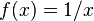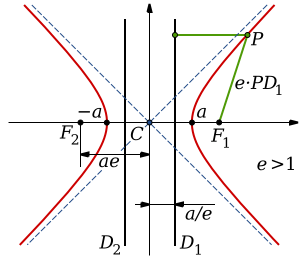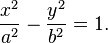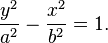# Hyperbola facts for kids

Kids Encyclopedia FactsA hyperbola is the intersection between both halves of a double cone and a plane.

A hyperbola is a type of conic section. Like the other three types of conic sections - parabolas, ellipses, and circles - it is a curve formed by the intersection of a cone and a plane. A hyperbola is created when the plane intersects both halves of a double cone, creating two curves that look exactly like each other, but open in opposite directions. This occurs when the angle between the axis of the cone and the plane is less than the angle between a line on the side of the cone and the plane.

Hyperbolas can be found in many places in nature. For example, an object in open orbit around another object - where it never returns - can move in the shape of a hyperbola. On a sundial, the path followed by the tip of the shadow over time is a hyperbola.

One of the most well-known hyperbolas is the graph of the equation$f(x) = 1/x$.

## Definitions and equationsGraph of a hyperbola (red curves). The asymptotes are shown as blue dashed lines. The center is labeled C and the two vertices are located at -a and a. The foci are labeled F1 and F2.

The two disconnected curves that make up a hyperbola are called arms or branches.

The two points where the branches are closest together are called the vertices. The line between these two points is called the transverse axis or major axis. The midpoint of the transverse axis is the center of the hyperbola.

At large distances from the center, the branches of the hyperbola approach two straight lines. These two lines are called the asymptotes. As the distance from the center increases, the hyperbola gets closer and closer to the asymptotes, but never intersects them.

The conjugate axis or minor axis is perpendicular, or at a right angle to, the transverse axis. The endpoints of the conjugate axis are at the height where a segment that intersects the vertex and is perpendicular to the transverse axis intersects the asymptotes.

A hyperbola that has a center at the origin of the Cartesian coordinate system, which is the point (0,0), and has a transverse axis on the x-axis can be written as the equation$\frac{x^{2}}{a^{2}} - \frac{y^{2}}{b^{2}} = 1.$

a is the distance between the center and a vertex. The length of the transverse axis is equal to 2a. b is the length of a perpendicular line segment from a vertex to an asymptote. The length of the conjugate axis is equal to 2b.

The two branches of the above type of hyperbola open to the left and the right. If the branches open up and down and the transverse axis is on the y-axis, then the hyperbola can be written as the equation$\frac{y^{2}}{a^{2}} - \frac{x^{2}}{b^{2}} = 1.$

## Hyperbolic trajectory

A hyperbolic trajectory is the trajectory followed by an object when its velocity is more than the escape velocity of a planet, satellite, or star. That means its orbital eccentricity is greater than 1. For example, meteors approach on a hyperbolic trajectory, and interplanetary space probes leave on one.

## Images for kidsHyperbola Facts for Kids. Kiddle Encyclopedia.